# RD Sharma Solutions for Class 10 Maths Chapter 1 Real Numbers Exercise 1.1

Real Numbers is the universal set containing natural numbers, integers, rational and irrational numbers. In RD Sharma Solutions Class 10 Exercise 1.1, we are going to understand the divisibility of integers and also some important properties of integers. Euclid’s division Lemma is the prime focus in this exercise. For an effective way of solving these questions students can download RD Sharma Solutions for Class 10 Maths Chapter 1 Real Numbers Exercise 1.1 PDF provided below.

## RD Sharma Solutions for Class 10 Maths Chapter 1 Real Numbers Exercise 1.1 Download PDF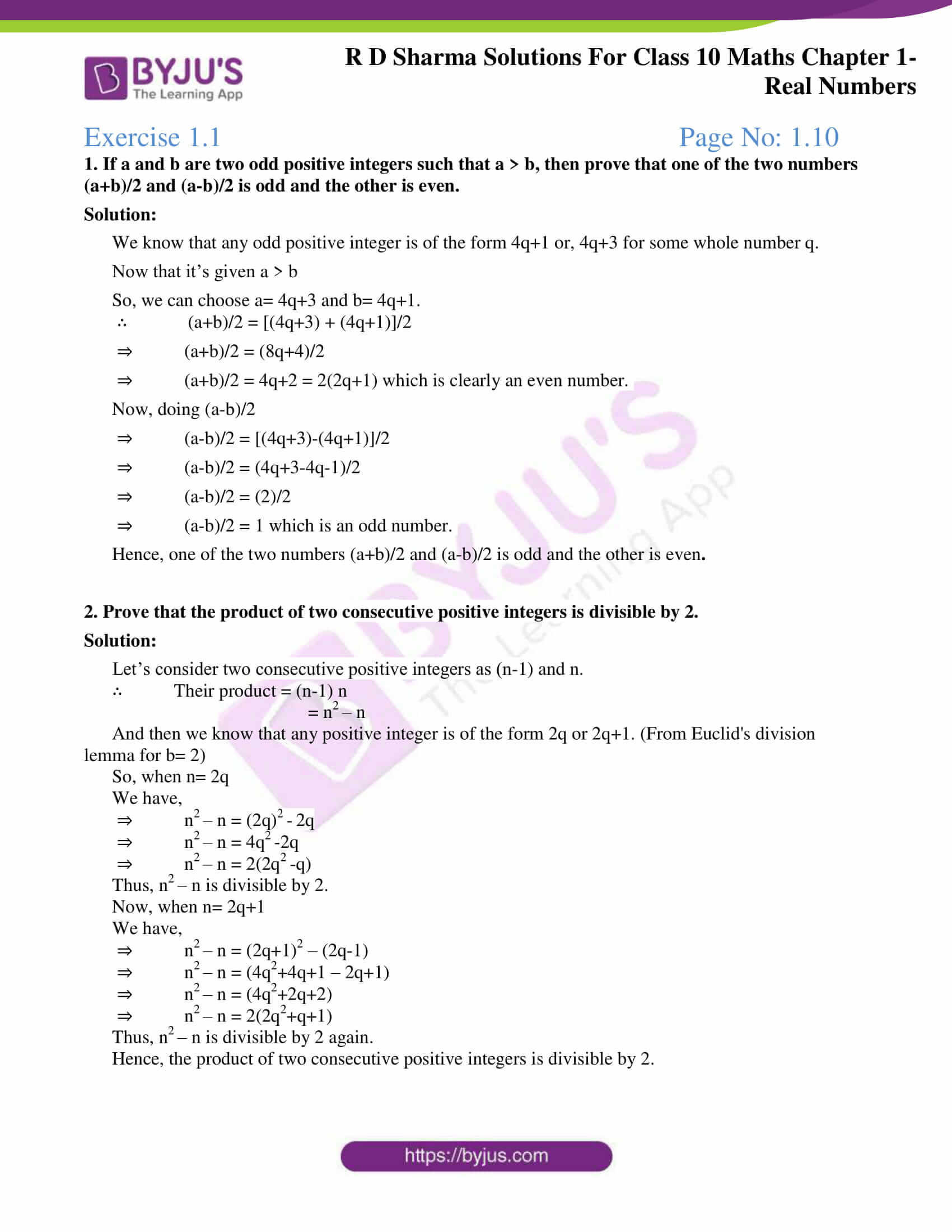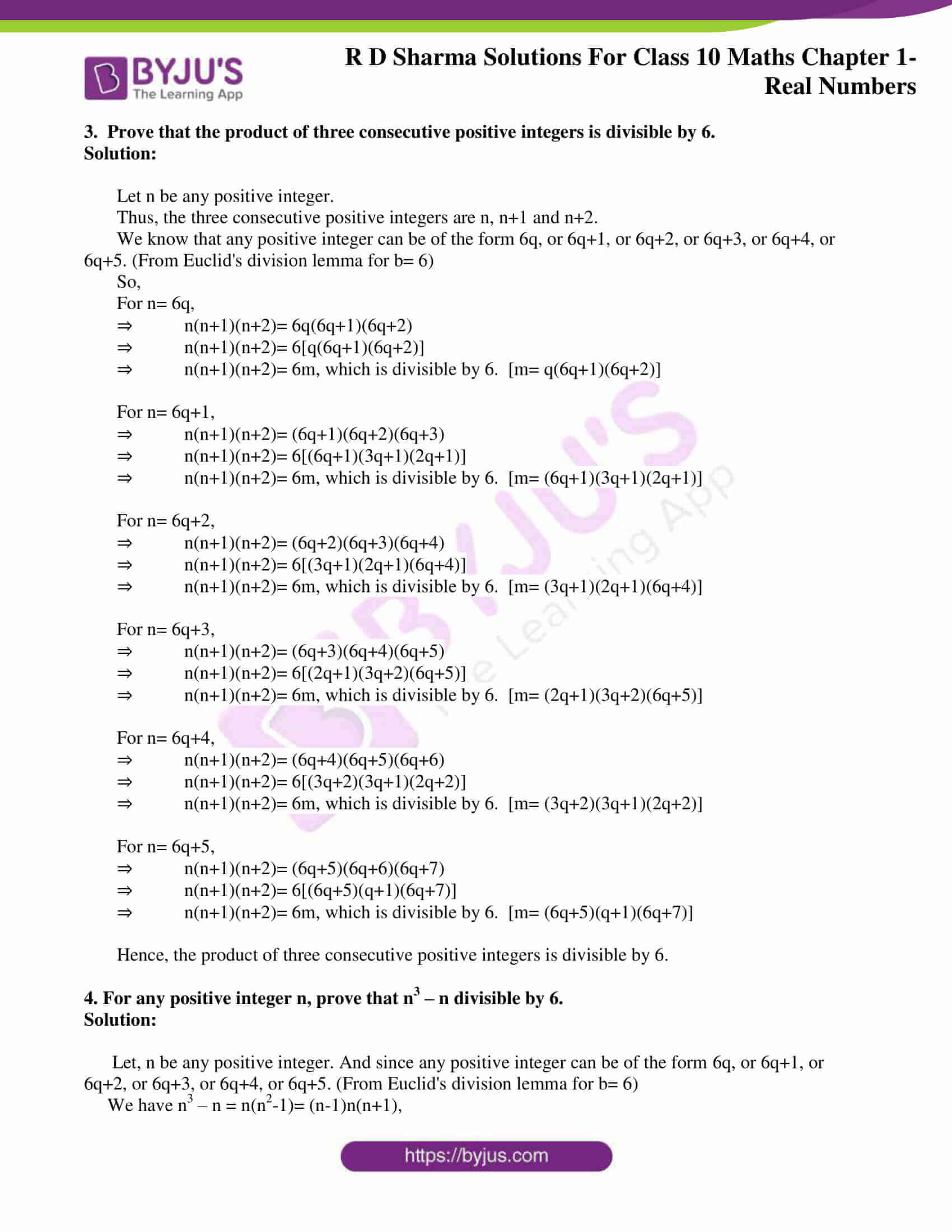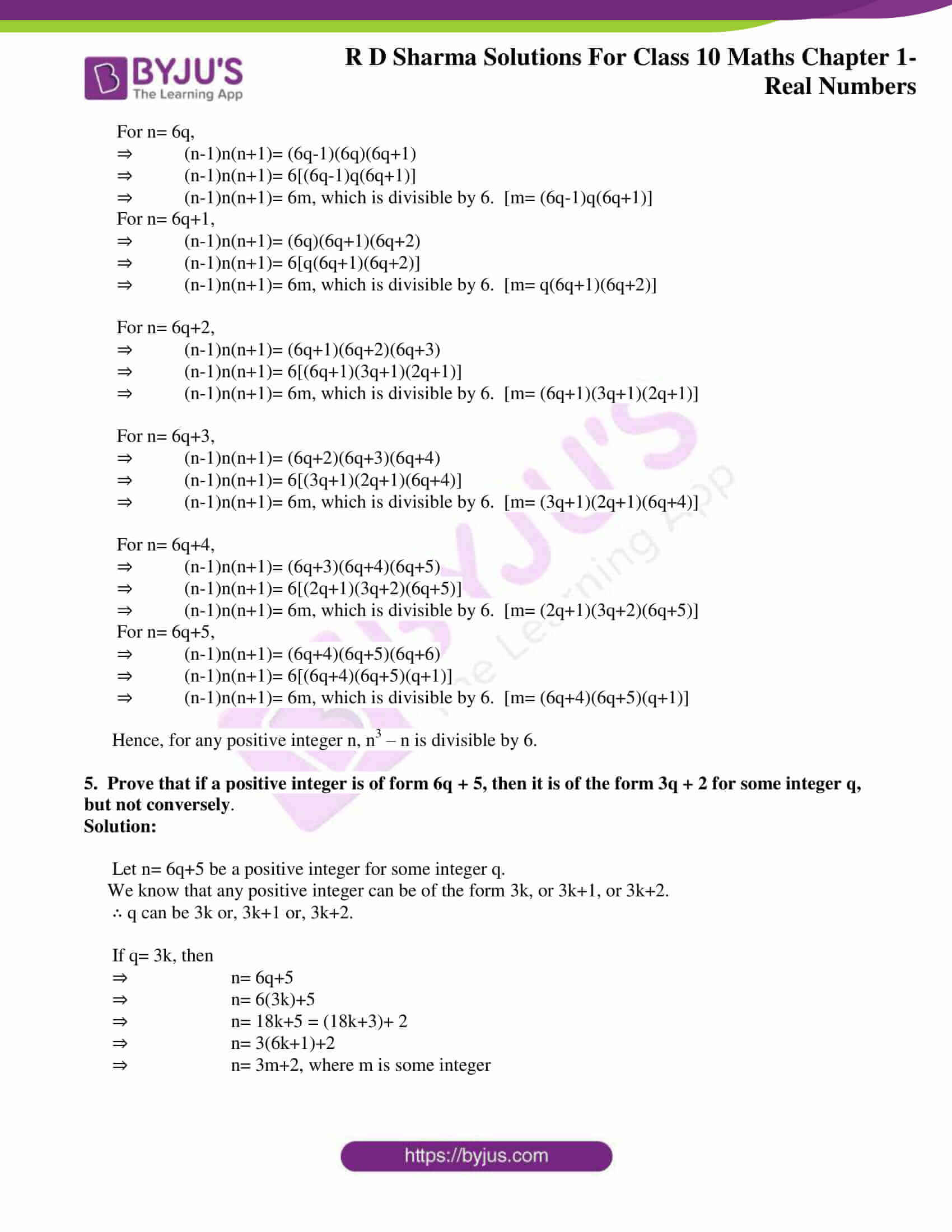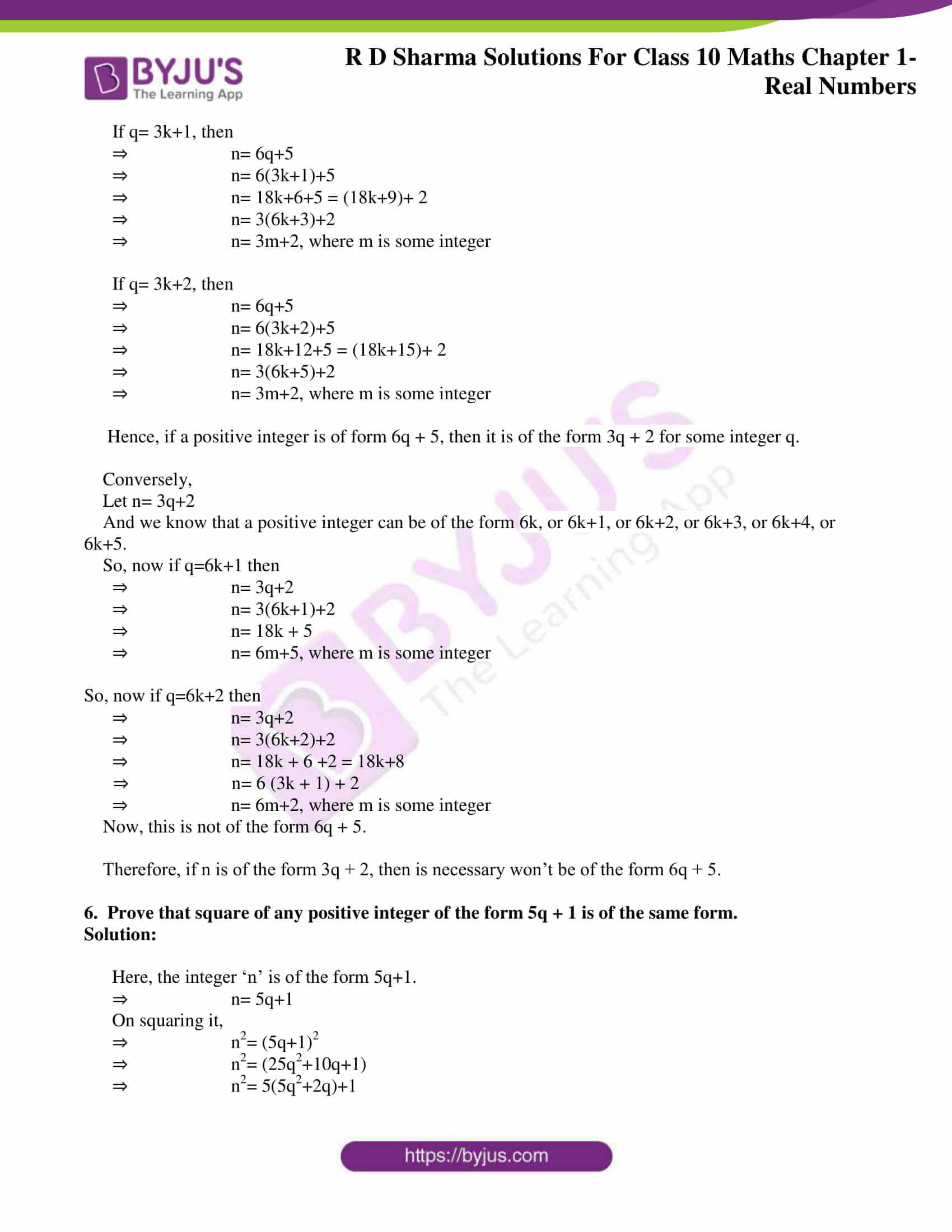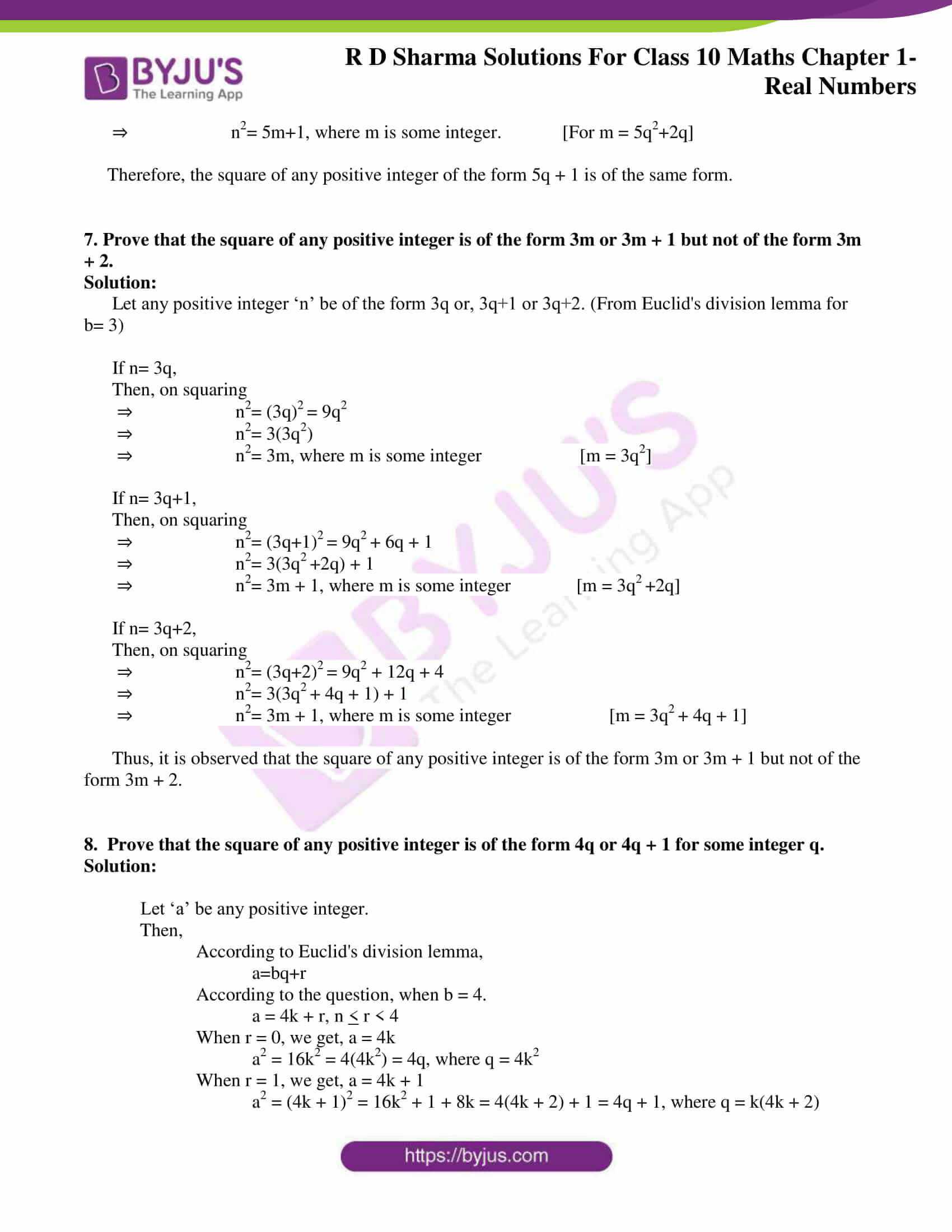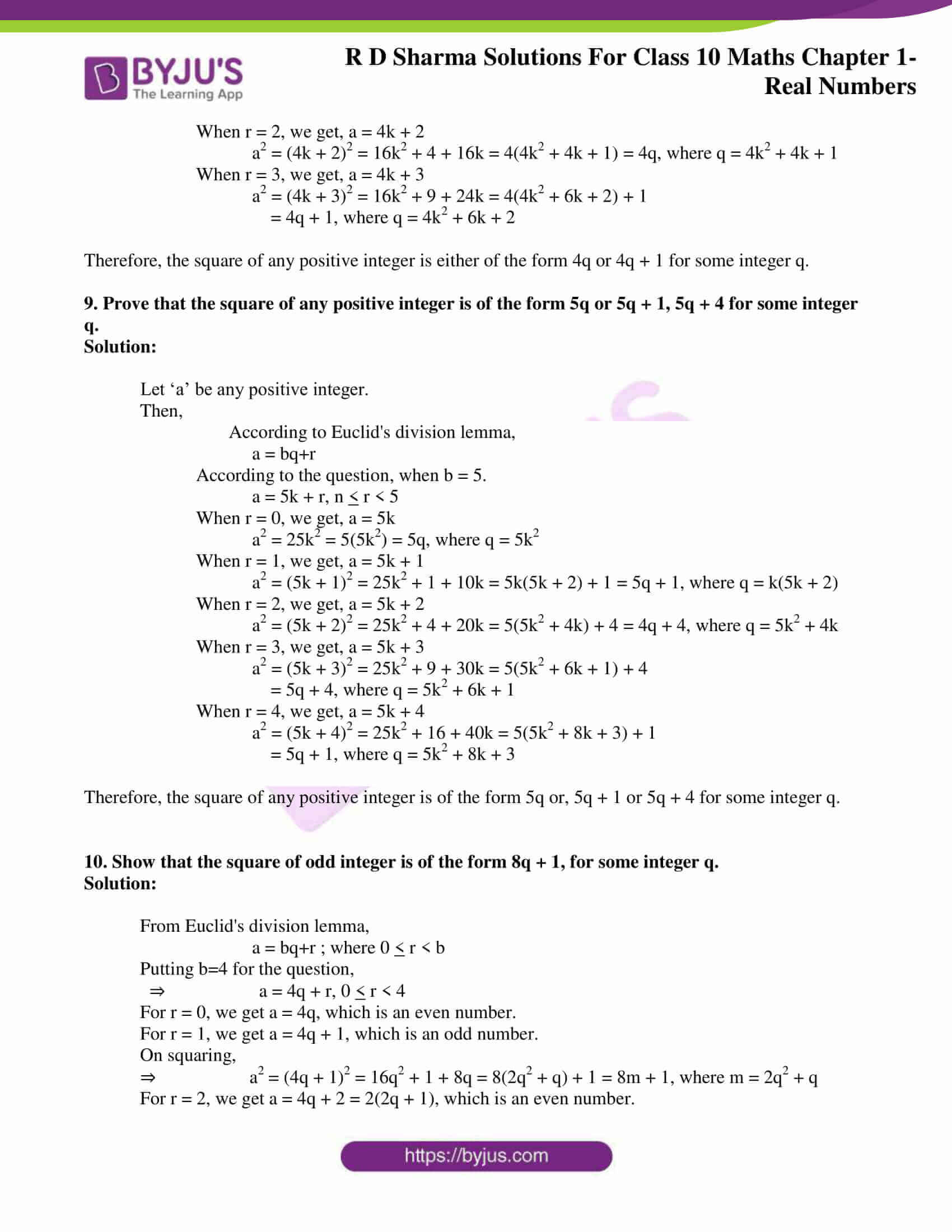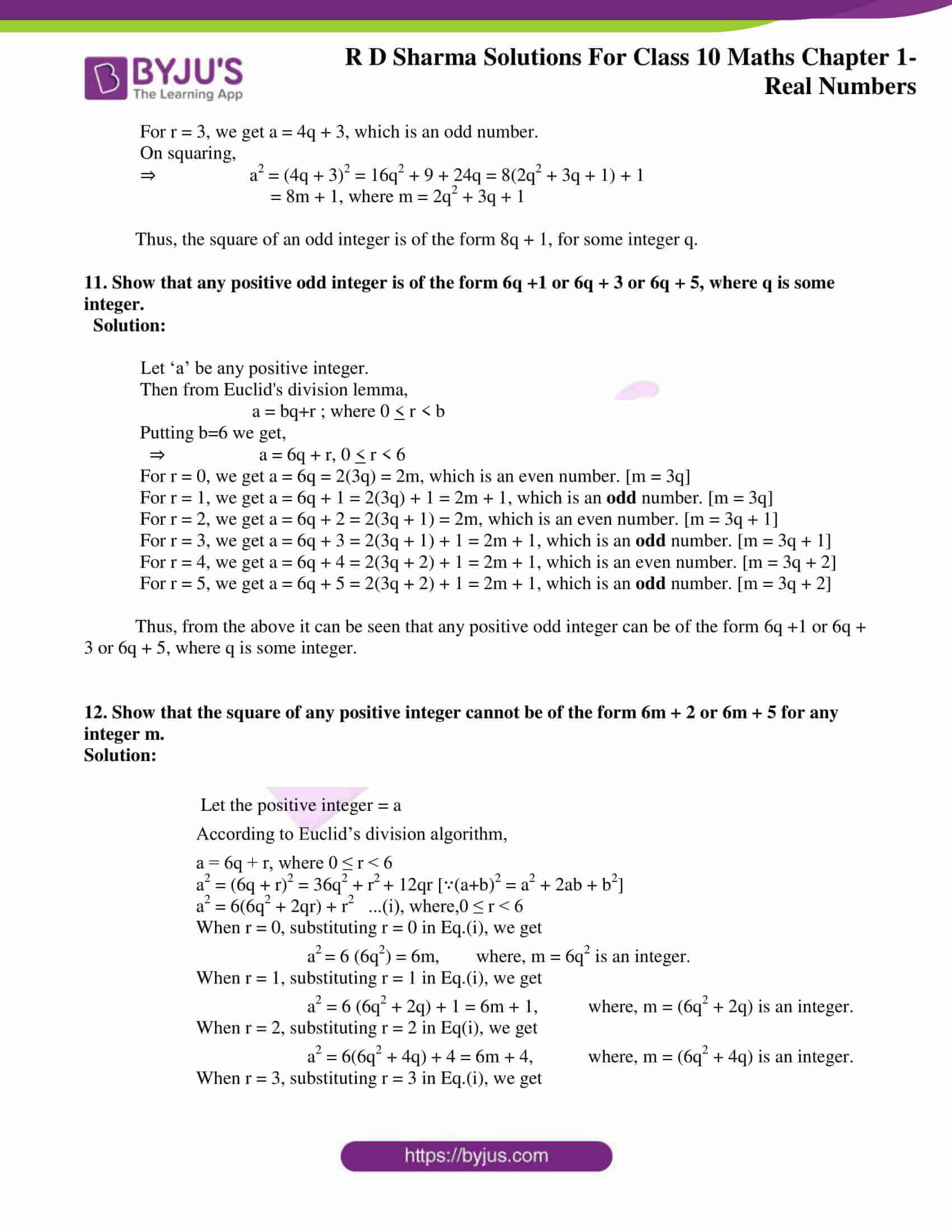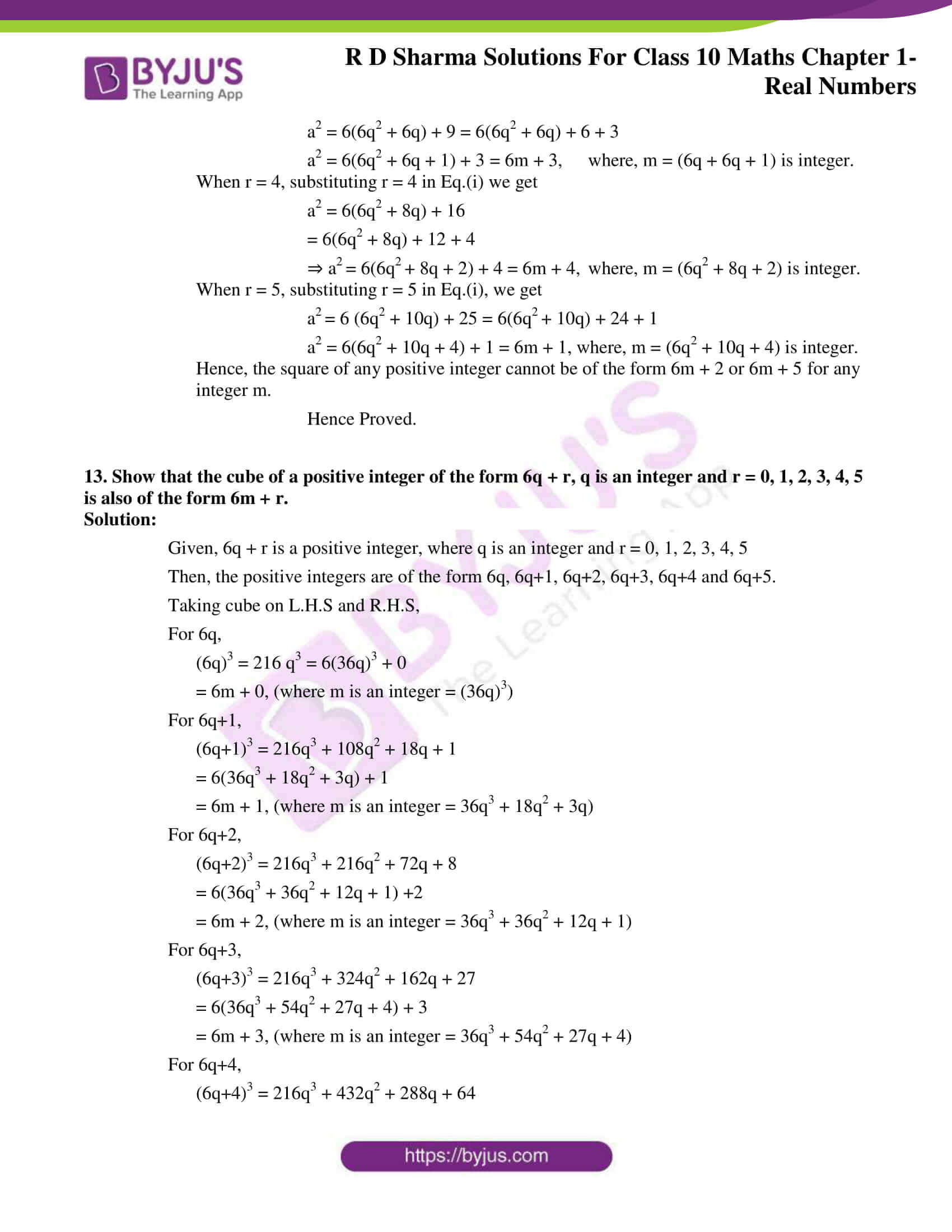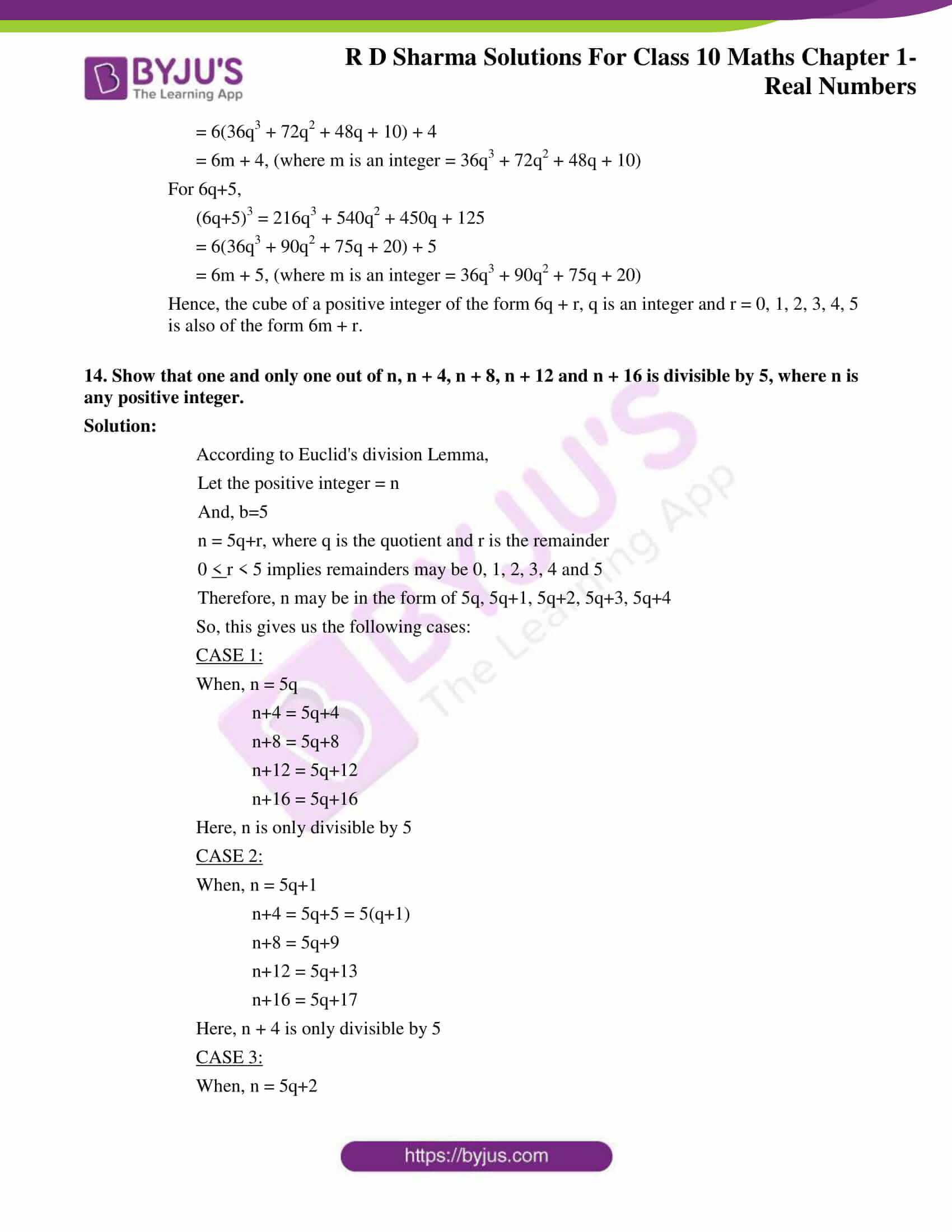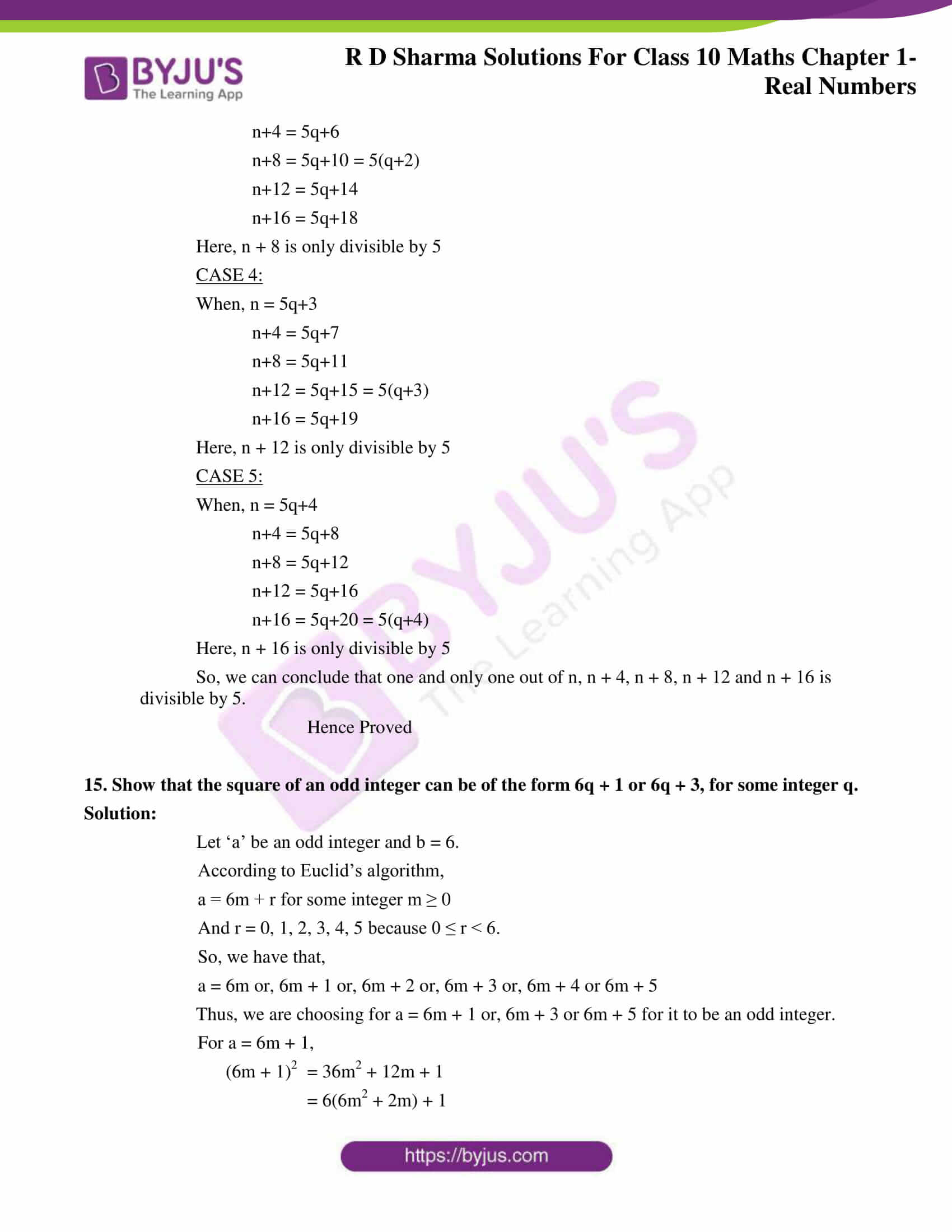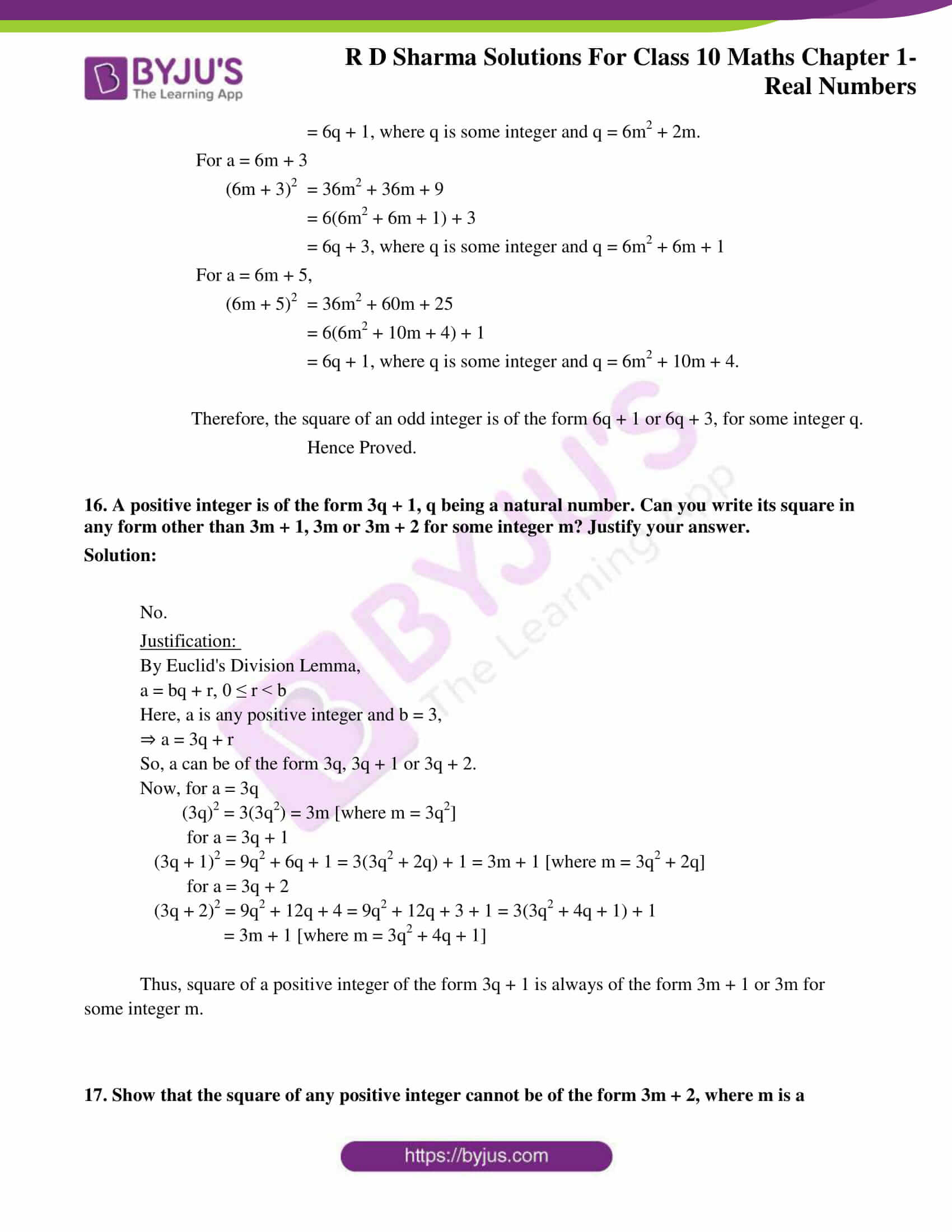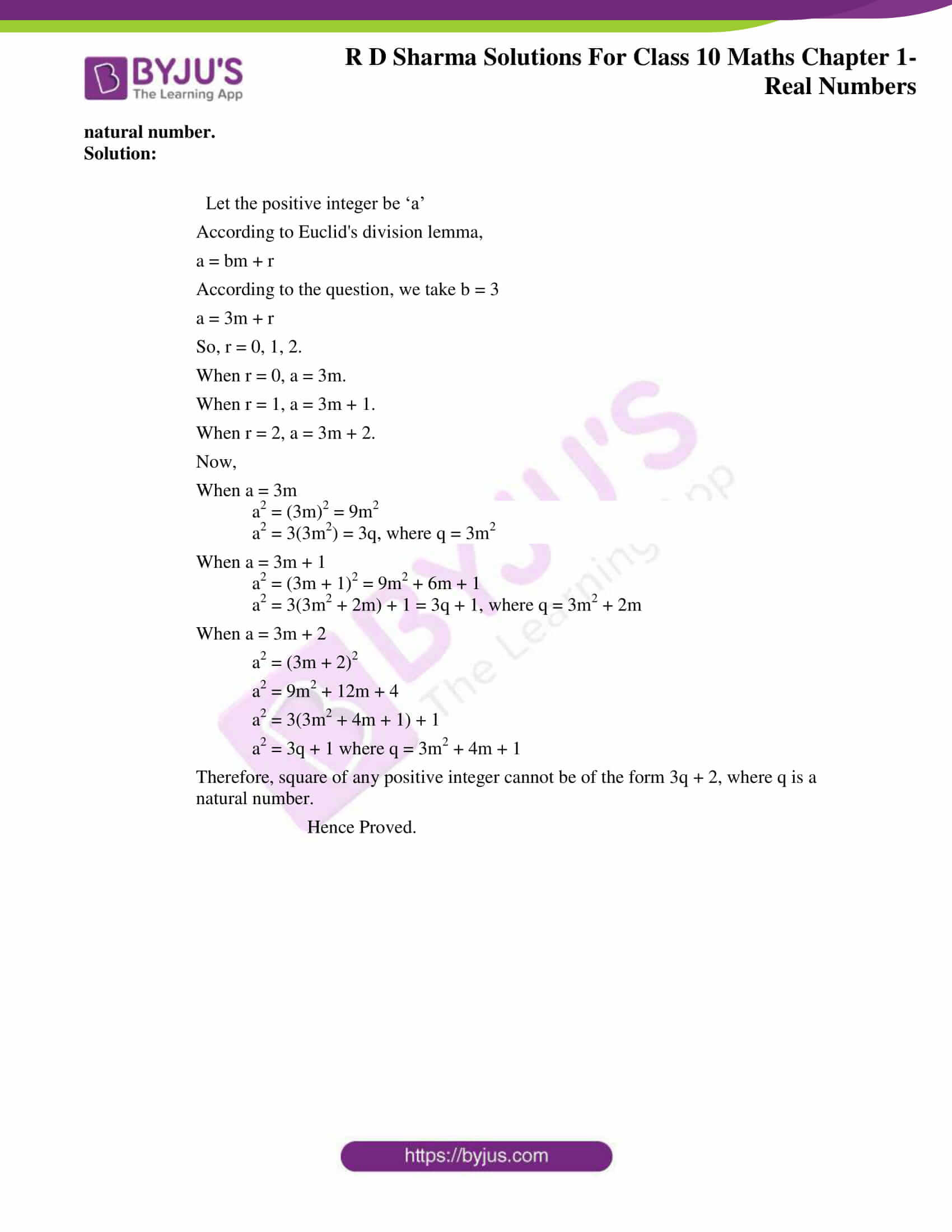### Access answers to Maths RD Sharma Solutions for Class 10 Chapter 1 Real Numbers Exercise 1.1

1. If a and b are two odd positive integers such that a > b, then prove that one of the two numbers (a+b)/2 and (a-b)/2 is odd and the other is even.

Solution:

We know that any odd positive integer is of the form 4q+1 or, 4q+3 for some whole number q.

Now that it’s given a > b

So, we can choose a= 4q+3 and b= 4q+1.

∴ (a+b)/2 = [(4q+3) + (4q+1)]/2

⇒ (a+b)/2 = (8q+4)/2

⇒ (a+b)/2 = 4q+2 = 2(2q+1) which is clearly an even number.

Now, doing (a-b)/2

⇒ (a-b)/2 = [(4q+3)-(4q+1)]/2

⇒ (a-b)/2 = (4q+3-4q-1)/2

⇒ (a-b)/2 = (2)/2

⇒ (a-b)/2 = 1 which is an odd number.

Hence, one of the two numbers (a+b)/2 and (a-b)/2 is odd and the other is even.

2. Prove that the product of two consecutive positive integers is divisible by 2.

Solution:

Let’s consider two consecutive positive integers as (n-1) and n.

∴ Their product = (n-1) n

= n2 – n

And then we know that any positive integer is of the form 2q or 2q+1. (From Euclid’s division lemma for b= 2)

So, when n= 2q

We have,

⇒ n2 – n = (2q)2 2q

⇒ n2 – n = 4q2 -2q

⇒ n2 – n = 2(2q2 -q)

Thus, n2 – n is divisible by 2.

Now, when n= 2q+1

We have,

⇒ n2 – n = (2q+1)2 – (2q-1)

⇒ n2 – n = (4q2+4q+1 – 2q+1)

⇒ n2 – n = (4q2+2q+2)

⇒ n2 – n = 2(2q2+q+1)

Thus, n2 – n is divisible by 2 again.

Hence, the product of two consecutive positive integers is divisible by 2.

3. Prove that the product of three consecutive positive integers is divisible by 6.

Solution:

Let n be any positive integer.

Thus, the three consecutive positive integers are n, n+1 and n+2.

We know that any positive integer can be of the form 6q, or 6q+1, or 6q+2, or 6q+3, or 6q+4, or 6q+5. (From Euclid’s division lemma for b= 6)

So,

For n= 6q,

⇒ n(n+1)(n+2)= 6q(6q+1)(6q+2)

⇒ n(n+1)(n+2)= 6[q(6q+1)(6q+2)]

⇒ n(n+1)(n+2)= 6m, which is divisible by 6. [m= q(6q+1)(6q+2)]

For n= 6q+1,

⇒ n(n+1)(n+2)= (6q+1)(6q+2)(6q+3)

⇒ n(n+1)(n+2)= 6[(6q+1)(3q+1)(2q+1)]

⇒ n(n+1)(n+2)= 6m, which is divisible by 6. [m= (6q+1)(3q+1)(2q+1)]

For n= 6q+2,

⇒ n(n+1)(n+2)= (6q+2)(6q+3)(6q+4)

⇒ n(n+1)(n+2)= 6[(3q+1)(2q+1)(6q+4)]

⇒ n(n+1)(n+2)= 6m, which is divisible by 6. [m= (3q+1)(2q+1)(6q+4)]

For n= 6q+3,

⇒ n(n+1)(n+2)= (6q+3)(6q+4)(6q+5)

⇒ n(n+1)(n+2)= 6[(2q+1)(3q+2)(6q+5)]

⇒ n(n+1)(n+2)= 6m, which is divisible by 6. [m= (2q+1)(3q+2)(6q+5)]

For n= 6q+4,

⇒ n(n+1)(n+2)= (6q+4)(6q+5)(6q+6)

⇒ n(n+1)(n+2)= 6[(3q+2)(3q+1)(2q+2)]

⇒ n(n+1)(n+2)= 6m, which is divisible by 6. [m= (3q+2)(3q+1)(2q+2)]

For n= 6q+5,

⇒ n(n+1)(n+2)= (6q+5)(6q+6)(6q+7)

⇒ n(n+1)(n+2)= 6[(6q+5)(q+1)(6q+7)]

⇒ n(n+1)(n+2)= 6m, which is divisible by 6. [m= (6q+5)(q+1)(6q+7)]

Hence, the product of three consecutive positive integers is divisible by 6.

4. For any positive integer n, prove that n3 – n divisible by 6.

Solution:

Let, n be any positive integer. And since any positive integer can be of the form 6q, or 6q+1, or 6q+2, or 6q+3, or 6q+4, or 6q+5. (From Euclid’s division lemma for b= 6)

We have n3 – n = n(n2-1)= (n-1)n(n+1),

For n= 6q,

⇒ (n-1)n(n+1)= (6q-1)(6q)(6q+1)

⇒ (n-1)n(n+1)= 6[(6q-1)q(6q+1)]

⇒ (n-1)n(n+1)= 6m, which is divisible by 6. [m= (6q-1)q(6q+1)]

For n= 6q+1,

⇒ (n-1)n(n+1)= (6q)(6q+1)(6q+2)

⇒ (n-1)n(n+1)= 6[q(6q+1)(6q+2)]

⇒ (n-1)n(n+1)= 6m, which is divisible by 6. [m= q(6q+1)(6q+2)]

For n= 6q+2,

⇒ (n-1)n(n+1)= (6q+1)(6q+2)(6q+3)

⇒ (n-1)n(n+1)= 6[(6q+1)(3q+1)(2q+1)]

⇒ (n-1)n(n+1)= 6m, which is divisible by 6. [m= (6q+1)(3q+1)(2q+1)]

For n= 6q+3,

⇒ (n-1)n(n+1)= (6q+2)(6q+3)(6q+4)

⇒ (n-1)n(n+1)= 6[(3q+1)(2q+1)(6q+4)]

⇒ (n-1)n(n+1)= 6m, which is divisible by 6. [m= (3q+1)(2q+1)(6q+4)]

For n= 6q+4,

⇒ (n-1)n(n+1)= (6q+3)(6q+4)(6q+5)

⇒ (n-1)n(n+1)= 6[(2q+1)(3q+2)(6q+5)]

⇒ (n-1)n(n+1)= 6m, which is divisible by 6. [m= (2q+1)(3q+2)(6q+5)]

For n= 6q+5,

⇒ (n-1)n(n+1)= (6q+4)(6q+5)(6q+6)

⇒ (n-1)n(n+1)= 6[(6q+4)(6q+5)(q+1)]

⇒ (n-1)n(n+1)= 6m, which is divisible by 6. [m= (6q+4)(6q+5)(q+1)]

Hence, for any positive integer n, n3 – n is divisible by 6.

5.  Prove that if a positive integer is of form 6q + 5, then it is of the form 3q + 2 for some integer q, but not conversely.

Solution:

Let n= 6q+5 be a positive integer for some integer q.

We know that any positive integer can be of the form 3k, or 3k+1, or 3k+2.

∴ q can be 3k or, 3k+1 or, 3k+2.

If q= 3k, then

⇒ n= 6q+5

⇒ n= 6(3k)+5

⇒ n= 18k+5 = (18k+3)+ 2

⇒ n= 3(6k+1)+2

⇒ n= 3m+2, where m is some integer

If q= 3k+1, then

⇒ n= 6q+5

⇒ n= 6(3k+1)+5

⇒ n= 18k+6+5 = (18k+9)+ 2

⇒ n= 3(6k+3)+2

⇒ n= 3m+2, where m is some integer

If q= 3k+2, then

⇒ n= 6q+5

⇒ n= 6(3k+2)+5

⇒ n= 18k+12+5 = (18k+15)+ 2

⇒ n= 3(6k+5)+2

⇒ n= 3m+2, where m is some integer

Hence, if a positive integer is of form 6q + 5, then it is of the form 3q + 2 for some integer q.

Conversely,

Let n= 3q+2

And we know that a positive integer can be of the form 6k, or 6k+1, or 6k+2, or 6k+3, or 6k+4, or 6k+5.

So, now if q=6k+1 then

⇒ n= 3q+2

⇒ n= 3(6k+1)+2

⇒ n= 18k + 5

⇒ n= 6m+5, where m is some integer

So, now if q=6k+2 then

⇒ n= 3q+2

⇒ n= 3(6k+2)+2

⇒ n= 18k + 6 +2 = 18k+8

⇒ n= 6 (3k + 1) + 2

⇒ n= 6m+2, where m is some integer

Now, this is not of the form 6q + 5.

Therefore, if n is of the form 3q + 2, then is necessary won’t be of the form 6q + 5.

6.  Prove that square of any positive integer of the form 5q + 1 is of the same form.

Solution:

Here, the integer ‘n’ is of the form 5q+1.

⇒ n= 5q+1

On squaring it,

⇒ n2= (5q+1)2

⇒ n2= (25q2+10q+1)

⇒ n2= 5(5q2+2q)+1

⇒ n2= 5m+1, where m is some integer. [For m = 5q2+2q]

Therefore, the square of any positive integer of the form 5q + 1 is of the same form.

7. Prove that the square of any positive integer is of the form 3m or 3m + 1 but not of the form 3m + 2.

Solution:

Let any positive integer ‘n’ be of the form 3q or, 3q+1 or 3q+2. (From Euclid’s division lemma for b= 3)

If n= 3q,

Then, on squaring

⇒ n2= (3q)2 = 9q2

⇒ n2= 3(3q2)

⇒ n2= 3m, where m is some integer [m = 3q2]

If n= 3q+1,

Then, on squaring

⇒ n2= (3q+1)2 = 9q2 + 6q + 1

⇒ n2= 3(3q2 +2q) + 1

⇒ n2= 3m + 1, where m is some integer [m = 3q2 +2q]

If n= 3q+2,

Then, on squaring

⇒ n2= (3q+2)2 = 9q2 + 12q + 4

⇒ n2= 3(3q2 + 4q + 1) + 1

⇒ n2= 3m + 1, where m is some integer [m = 3q2 + 4q + 1]

Thus, it is observed that the square of any positive integer is of the form 3m or 3m + 1 but not of the form 3m + 2.

8.  Prove that the square of any positive integer is of the form 4q or 4q + 1 for some integer q.

Solution:

Let ‘a’ be any positive integer.

Then,

According to Euclid’s division lemma,

a=bq+r

According to the question, when b = 4.

a = 4k + r, n < r < 4

When r = 0, we get, a = 4k

a2 = 16k2 = 4(4k2) = 4q, where q = 4k2

When r = 1, we get, a = 4k + 1

a2 = (4k + 1)2 = 16k2 + 1 + 8k = 4(4k + 2) + 1 = 4q + 1, where q = k(4k + 2)

When r = 2, we get, a = 4k + 2

a2 = (4k + 2)2 = 16k2 + 4 + 16k = 4(4k2 + 4k + 1) = 4q, where q = 4k2 + 4k + 1

When r = 3, we get, a = 4k + 3

a2 = (4k + 3)2 = 16k2 + 9 + 24k = 4(4k2 + 6k + 2) + 1

= 4q + 1, where q = 4k2 + 6k + 2

Therefore, the square of any positive integer is either of the form 4q or 4q + 1 for some integer q.

9. Prove that the square of any positive integer is of the form 5q or 5q + 1, 5q + 4 for some integer q.

Solution:

Let ‘a’ be any positive integer.

Then,

According to Euclid’s division lemma,

a = bq+r

According to the question, when b = 5.

a = 5k + r, n < r < 5

When r = 0, we get, a = 5k

a2 = 25k2 = 5(5k2) = 5q, where q = 5k2

When r = 1, we get, a = 5k + 1

a2 = (5k + 1)2 = 25k2 + 1 + 10k = 5k(5k + 2) + 1 = 5q + 1, where q = k(5k + 2)

When r = 2, we get, a = 5k + 2

a2 = (5k + 2)2 = 25k2 + 4 + 20k = 5(5k2 + 4k) + 4 = 4q + 4, where q = 5k2 + 4k

When r = 3, we get, a = 5k + 3

a2 = (5k + 3)2 = 25k2 + 9 + 30k = 5(5k2 + 6k + 1) + 4

= 5q + 4, where q = 5k2 + 6k + 1

When r = 4, we get, a = 5k + 4

a2 = (5k + 4)2 = 25k2 + 16 + 40k = 5(5k2 + 8k + 3) + 1

= 5q + 1, where q = 5k2 + 8k + 3

Therefore, the square of any positive integer is of the form 5q or, 5q + 1 or 5q + 4 for some integer q.

10. Show that the square of odd integer is of the form 8q + 1, for some integer q.

Solution:

From Euclid’s division lemma,

a = bq+r ; where 0 < r < b

Putting b=4 for the question,

⇒ a = 4q + r, 0 < r < 4

For r = 0, we get a = 4q, which is an even number.

For r = 1, we get a = 4q + 1, which is an odd number.

On squaring,

⇒ a2 = (4q + 1)2 = 16q2 + 1 + 8q = 8(2q2 + q) + 1 = 8m + 1, where m = 2q2 + q

For r = 2, we get a = 4q + 2 = 2(2q + 1), which is an even number.

For r = 3, we get a = 4q + 3, which is an odd number.

On squaring,

⇒ a2 = (4q + 3)2 = 16q2 + 9 + 24q = 8(2q2 + 3q + 1) + 1

= 8m + 1, where m = 2q2 + 3q + 1

Thus, the square of an odd integer is of the form 8q + 1, for some integer q.

11. Show that any positive odd integer is of the form 6q +1 or 6q + 3 or 6q + 5, where q is some integer.

Solution:

Let ‘a’ be any positive integer.

Then from Euclid’s division lemma,

a = bq+r ; where 0 < r < b

Putting b=6 we get,

⇒ a = 6q + r, 0 < r < 6

For r = 0, we get a = 6q = 2(3q) = 2m, which is an even number. [m = 3q]

For r = 1, we get a = 6q + 1 = 2(3q) + 1 = 2m + 1, which is an odd number. [m = 3q]

For r = 2, we get a = 6q + 2 = 2(3q + 1) = 2m, which is an even number. [m = 3q + 1]

For r = 3, we get a = 6q + 3 = 2(3q + 1) + 1 = 2m + 1, which is an odd number. [m = 3q + 1]

For r = 4, we get a = 6q + 4 = 2(3q + 2) + 1 = 2m + 1, which is an even number. [m = 3q + 2]

For r = 5, we get a = 6q + 5 = 2(3q + 2) + 1 = 2m + 1, which is an odd number. [m = 3q + 2]

Thus, from the above it can be seen that any positive odd integer can be of the form 6q +1 or 6q + 3 or 6q + 5, where q is some integer.

12. Show that the square of any positive integer cannot be of the form 6m + 2 or 6m + 5 for any integer m.

Solution:

Let the positive integer = a

According to Euclid’s division algorithm,

a = 6q + r, where 0 ≤ r < 6

a2 = (6q + r)2 = 36q2 + r+ 12qr [∵(a+b)2 = a2 + 2ab + b2]
a2 = 6(6q2 + 2qr) + r2   …(i), where,0 ≤ r < 6

When r = 0, substituting r = 0 in Eq.(i), we get

a= 6 (6q2) = 6m, where, m = 6q2 is an integer.

When r = 1, substituting r = 1 in Eq.(i), we get

a2 = 6 (6q2 + 2q) + 1 = 6m + 1, where, m = (6q2 + 2q) is an integer.

When r = 2, substituting r = 2 in Eq(i), we get

a2 = 6(6q2 + 4q) + 4 = 6m + 4, where, m = (6q2 + 4q) is an integer.

When r = 3, substituting r = 3 in Eq.(i), we get

a2 = 6(6q2 + 6q) + 9 = 6(6q2 + 6q) + 6 + 3

a2 = 6(6q2 + 6q + 1) + 3 = 6m + 3, where, m = (6q + 6q + 1) is integer.

When r = 4, substituting r = 4 in Eq.(i) we get

a2 = 6(6q2 + 8q) + 16

= 6(6q2 + 8q) + 12 + 4

⇒ a= 6(6q+ 8q + 2) + 4 = 6m + 4, where, m = (6q2 + 8q + 2) is integer.

When r = 5, substituting r = 5 in Eq.(i), we get

a= 6 (6q2 + 10q) + 25 = 6(6q+ 10q) + 24 + 1

a2 = 6(6q2 + 10q + 4) + 1 = 6m + 1, where, m = (6q2 + 10q + 4) is integer.

Hence, the square of any positive integer cannot be of the form 6m + 2 or 6m + 5 for any integer m.

Hence Proved.

13. Show that the cube of a positive integer of the form 6q + r, q is an integer and r = 0, 1, 2, 3, 4, 5 is also of the form 6m + r.

Solution:

Given, 6q + r is a positive integer, where q is an integer and r = 0, 1, 2, 3, 4, 5

Then, the positive integers are of the form 6q, 6q+1, 6q+2, 6q+3, 6q+4 and 6q+5.

Taking cube on L.H.S and R.H.S,

For 6q,

(6q)3 = 216 q3 = 6(36q)3 + 0

= 6m + 0, (where m is an integer = (36q)3)

For 6q+1,

(6q+1)3 = 216q3 + 108q2 + 18q + 1

= 6(36q3 + 18q2 + 3q) + 1

= 6m + 1, (where m is an integer = 36q3 + 18q2 + 3q)

For 6q+2,

(6q+2)3 = 216q3 + 216q2 + 72q + 8

= 6(36q3 + 36q2 + 12q + 1) +2

= 6m + 2, (where m is an integer = 36q3 + 36q2 + 12q + 1)

For 6q+3,

(6q+3)3 = 216q3 + 324q2 + 162q + 27

= 6(36q3 + 54q2 + 27q + 4) + 3

= 6m + 3, (where m is an integer = 36q3 + 54q2 + 27q + 4)

For 6q+4,

(6q+4)3 = 216q3 + 432q2 + 288q + 64

= 6(36q3 + 72q2 + 48q + 10) + 4

= 6m + 4, (where m is an integer = 36q3 + 72q2 + 48q + 10)

For 6q+5,

(6q+5)3 = 216q3 + 540q2 + 450q + 125

= 6(36q3 + 90q2 + 75q + 20) + 5

= 6m + 5, (where m is an integer = 36q3 + 90q2 + 75q + 20)

Hence, the cube of a positive integer of the form 6q + r, q is an integer and r = 0, 1, 2, 3, 4, 5 is also of the form 6m + r.

14. Show that one and only one out of n, n + 4, n + 8, n + 12 and n + 16 is divisible by 5, where n is any positive integer.

Solution:

According to Euclid’s division Lemma,

Let the positive integer = n

And, b=5

n = 5q+r, where q is the quotient and r is the remainder

0 < r < 5 implies remainders may be 0, 1, 2, 3, 4 and 5

Therefore, n may be in the form of 5q, 5q+1, 5q+2, 5q+3, 5q+4

So, this gives us the following cases:

CASE 1:

When, n = 5q

n+4 = 5q+4

n+8 = 5q+8

n+12 = 5q+12

n+16 = 5q+16

Here, n is only divisible by 5

CASE 2:

When, n = 5q+1

n+4 = 5q+5 = 5(q+1)

n+8 = 5q+9

n+12 = 5q+13

n+16 = 5q+17

Here, n + 4 is only divisible by 5

CASE 3:

When, n = 5q+2

n+4 = 5q+6

n+8 = 5q+10 = 5(q+2)

n+12 = 5q+14

n+16 = 5q+18

Here, n + 8 is only divisible by 5

CASE 4:

When, n = 5q+3

n+4 = 5q+7

n+8 = 5q+11

n+12 = 5q+15 = 5(q+3)

n+16 = 5q+19

Here, n + 12 is only divisible by 5

CASE 5:

When, n = 5q+4

n+4 = 5q+8

n+8 = 5q+12

n+12 = 5q+16

n+16 = 5q+20 = 5(q+4)

Here, n + 16 is only divisible by 5

So, we can conclude that one and only one out of n, n + 4, n + 8, n + 12 and n + 16 is divisible by 5.

Hence Proved

15. Show that the square of an odd integer can be of the form 6q + 1 or 6q + 3, for some integer q.

Solution:

Let ‘a’ be an odd integer and b = 6.

According to Euclid’s algorithm,

a = 6m + r for some integer m ≥ 0

And r = 0, 1, 2, 3, 4, 5 because 0 ≤ r < 6.

So, we have that,

a = 6m or, 6m + 1 or, 6m + 2 or, 6m + 3 or, 6m + 4 or 6m + 5

Thus, we are choosing for a = 6m + 1 or, 6m + 3 or 6m + 5 for it to be an odd integer.

For a = 6m + 1,

(6m + 1)2 = 36m2 + 12m + 1

= 6(6m2 + 2m) + 1

= 6q + 1, where q is some integer and q = 6m2 + 2m.

For a = 6m + 3

(6m + 3)2 = 36m2 + 36m + 9

= 6(6m2 + 6m + 1) + 3

= 6q + 3, where q is some integer and q = 6m2 + 6m + 1

For a = 6m + 5,

(6m + 5)2 = 36m2 + 60m + 25

= 6(6m2 + 10m + 4) + 1

= 6q + 1, where q is some integer and q = 6m2 + 10m + 4.

Therefore, the square of an odd integer is of the form 6q + 1 or 6q + 3, for some integer q.

Hence Proved.

16. A positive integer is of the form 3q + 1, q being a natural number. Can you write its square in any form other than 3m + 1, 3m or 3m + 2 for some integer m? Justify your answer.

Solution:

No.

Justification:

By Euclid’s Division Lemma,

a = bq + r, 0 ≤ r < b

Here, a is any positive integer and b = 3,

⇒ a = 3q + r

So, a can be of the form 3q, 3q + 1 or 3q + 2.

Now, for a = 3q

(3q)2 = 3(3q2) = 3m [where m = 3q2]

for a = 3q + 1

(3q + 1)2 = 9q2 + 6q + 1 = 3(3q2 + 2q) + 1 = 3m + 1 [where m = 3q2 + 2q]

for a = 3q + 2

(3q + 2)2 = 9q2 + 12q + 4 = 9q2 + 12q + 3 + 1 = 3(3q2 + 4q + 1) + 1

= 3m + 1 [where m = 3q2 + 4q + 1]

Thus, square of a positive integer of the form 3q + 1 is always of the form 3m + 1 or 3m for some integer m.

17. Show that the square of any positive integer cannot be of the form 3m + 2, where m is a natural number.

Solution:

Let the positive integer be ‘a’

According to Euclid’s division lemma,

a = bm + r

According to the question, we take b = 3

a = 3m + r

So, r = 0, 1, 2.

When r = 0, a = 3m.

When r = 1, a = 3m + 1.

When r = 2, a = 3m + 2.

Now,

When a = 3m

a2 = (3m)2 = 9m2

a2 = 3(3m2) = 3q, where q = 3m2

When a = 3m + 1

a2 = (3m + 1)2 = 9m2 + 6m + 1

a2 = 3(3m2 + 2m) + 1 = 3q + 1, where q = 3m2 + 2m

When a = 3m + 2

a2 = (3m + 2)2

a2 = 9m2 + 12m + 4

a2 = 3(3m2 + 4m + 1) + 1

a2 = 3q + 1 where q = 3m2 + 4m + 1

Therefore, square of any positive integer cannot be of the form 3q + 2, where q is a natural number.

Hence Proved.